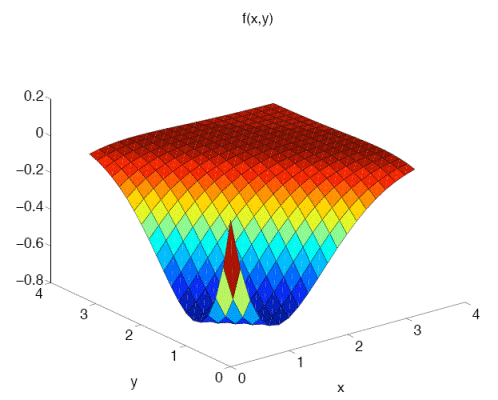# Tip: Using surf and meshgrid to create surface plots

https://www.cac.cornell.edu/vw/matlab/tips7.aspx

To complement its two-dimensional line plots, MATLAB also provides the ‘surf’ function for making three-dimensional (surface) plots. Plots of this sort are useful in many areas of science and engineering, in particular for making diagrams and teaching simple concepts in optimization and vector calculus. Unfortunately, creating surface plots has not always been completely straightforward in MATLAB, and actually required the helper function ‘meshgrid’ in order to work correctly. Newer versions have ameliorated some of this difficulty by accepting vector input arguments, but here we will describe the “classic” way of making surface plots in MATLAB.

Below we create a function f(x,y) composed of sinusoids and exponentials, which also happens to be symmetric in x and y, to illustrate the process of creating a surface plot in MATLAB. We plot this smooth example function on the square domain [0,pi]^2, using ‘N1′ points in the x-direction and ‘N2′ points in y. In this particular case, N1=N2=20 but in general they need not match. After creating the one-dimensional arrays x_array and y_array, we then proceed to loop over them with nested for’s, and fill in the values of the smooth function we plan to plot.

N1 = 20;
N2 = 20;
x_array = linspace(0, pi, N1);
y_array = linspace(0, pi, N2);

for n=1:N1
for m=1:N2
x = x_array(n);
y = y_array(m);
f(n,m) = -2*exp(-x)*exp(-y)* …
(sin(y)*cos(x) + cos(y)*sin(x));
end
end

Since some forms of the ‘surf’ command take three matrix arguments as input, we must perform an intermediate processing step on x_array and y_array before passing them to the ‘surf’ function. (Note: x_array and y_array can be passed directly in newer versions of MATLAB.) This intermediate processing step is handled by the ‘meshgrid’ command, which takes two vectors as input and returns two matrices in the format required by surf. The surf format is essentially a matrix X with the x_array values repeated row-wise and a matrix Y with the y_array values repeated column-wise.

We then call the ‘surf’ command and pass f’ (the transpose of f) instead of f, since by default the surf function plots ordered pairs (x(j), y(i), f(i,j)) rather than (x(i), y(j), f(i,j)). Finally, in addition to some simple labels, we also set the default three-dimensional view by calling ‘view(3)’. Note that the view command can also be used to set the azimuthal (AZ) and elevation (EL) angles directly, but the default three-dimensional view, AZ=-37.5, EL=30 is sufficient for this example. The resulting three-dimensional plot, when printed as a PDF, is sharp-looking and scales well for presentation on any size document or display device.

[X,Y] = meshgrid(x_array,y_array);
surf(X,Y,f’);
view(3);
xh=xlabel(‘x’);
yh=ylabel(‘y’);
th=title(‘f(x,y)’);